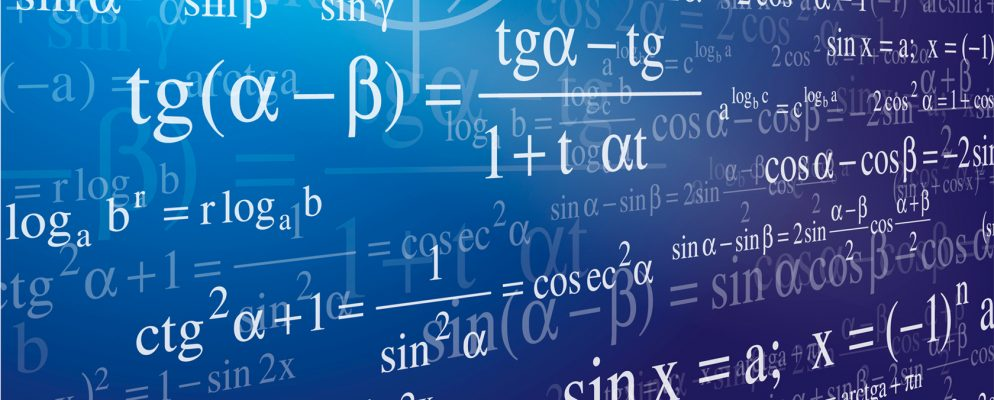# BEAMA Indices|Worked Example

### FormulaIf the cost to the Contractor of performing his obligations under the Contract shall be increased or reduced by reason of any rise or fall in labour costs or in the cost of material the amount of such increase or reduction shall be added to or deducted from the Contract Price as the case may be. Provided that no account shall be taken of any amount by which any cost incurred by the Contractor has been increased by the default or negligence of the Contractor.
Variations in the cost of materials and labour shall be calculated in accordance with the following Formula:
$$P_n = \frac{P_0}{100} \left(5 +47.5\times \frac{{BEL}_{avg|t:33\rightarrow100}}{{BEL}_0} + 47.5\times \frac{{BEE}_{avg|t:40\rightarrow80}}{{BEE}_0} \right)$$where
$$P_0$$ = Contract Price at Date of Tender

$$P_n$$ = Final Contract Price

$${BEE}_0$$ = BEAMA Electrical Equipment index figure last published before the date of tender

$${BEE}_{avg|t:40\rightarrow80}$$ = Average of BEAMA Electrical Equipment index last published at $$\frac{2}{5}$$ point of the Contract Period and ending with the Index last published before the $$\frac{4}{5}$$ point of the Contract Period.

$${BEL}_0$$ = BEAMA Labour Cost index figure for Electrical Engineering published for the month in which the tender date falls.

$${BEL}_{avg|t:33\rightarrow100}$$ = Average of the BEAMA Labour Cost index figures for Electrical Engineering published for the last $$\frac{2}{3}$$ of the Contract Period

### Data

• A - Contract Price,  $$P_0$$ = £100,000
• B - Date of Tender,  $$T_0$$ = 20 Jan 2016
• C - Date of Order,   $$T_1$$ = 01 Apr 2016
• D - Completion Date,  $$T_2$$ = 31 Mar 2019

### Calculation

#### Contract Days

• E - Contract days between C  and D, $$\Delta T = T_2 - T_1 = 1094$$

#### Electrical Labour Indices (Jan 2010=100)

• F - Date at one-third of Contract Period, $$T_{33\%} = T_1+\frac{1}{3}\Delta T = T_1 + 365(days)$$ = (31/Mar/2017)
• G - Completion date, $$T_{100\%} = T_2 =$$ (31/Mar/2019)
• H - Average of indices published between F and G, $${BEL}_{avg|t:33\rightarrow100} =$$ avg(issue$$_{571}$$ to issue$$_{595}$$) = $$\frac{1}{595-571+1}\sum\limits_{n=571}^{595} I_n$$ = 122.1
• I - Index published for the month in which the tender date falls B, $${BEL}_0$$ = issue$$_{556}$$ = $$I_{556}$$ = 114.8

#### Electrical Material Indices (2010=100)

• J - Date at two-fifths of Contract Period, $$T_{40\%} = T_1+\frac{2}5\Delta T = T_1 + 438(days)$$ = (12/Jun/2017)
• K - Date at four-fifths of Contract Period, $$T_{80\%} = T_1+\frac{4}{5}\Delta T = T_1 + 875(days)$$ = (24/Aug/2018)
• L - Average of indices published between J and K, $${BEE}_{avg|t:40\rightarrow80} =$$ avg(issue$$_{573}$$ to issue$$_{588}$$) = $$\frac{1}{588-573+1}\sum\limits_{n=573}^{588} I_n$$ = 109.2
• M - Index last published before the date of tender B, $${BEE}_0$$ = issue$$_{557}$$ = $$I_{557}$$ = 93.1

#### Final Contract Price

$$\begin{eqnarray*} P_n &=& \frac{P_0}{100} \left(5 +47.5\times \frac{{BEL}_{avg|t:33\rightarrow100}}{{BEL}_0} + 47.5\times \frac{{BEE}_{avg|t:40\rightarrow80}}{{BEE}_0} \right)\\ &=& \frac{100,000}{100} \left[5+47.5\times \frac{122.1}{114.8}+47.5\times \frac{109.2}{93.1} \right] \\ &=& 111,231.81 \end{eqnarray*}$$

$$\begin{eqnarray*} \Delta P &=& P_n - P_0 \\ &=& 111,231.81 - 100,000.00 \\ &=& 11,231.81 \end{eqnarray*}$$